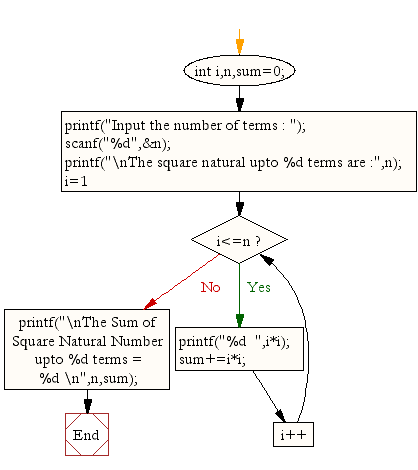﻿ C : Calculate n terms of square natural number and their sum# C Exercises: Calculate n terms of square natural number and their sum

## C For Loop: Exercise-25 with Solution

Write a program in C to display the n terms of square natural number and their sum. The series is as below:
1 4 9 16 ... n Terms

Sample Solution:

C Code:

``````#include <stdio.h>

void main()
{
int i,n,sum=0;
printf("Input the number of terms : ");
scanf("%d",&n);
printf("\nThe square natural upto %d terms are :",n);
for(i=1;i<=n;i++)
{
printf("%d  ",i*i);
sum+=i*i;
}
printf("\nThe Sum of Square Natural Number upto %d terms = %d \n",n,sum);
}
```
```

Sample Output:

```Input the number of terms : 5

The square natural upto 5 terms are :1  4  9  16  25
The Sum of Square Natural Number upto 5 terms = 55
```

Flowchart:C Programming Code Editor:

Improve this sample solution and post your code through Disqus.

What is the difficulty level of this exercise?

Test your Programming skills with w3resource's quiz.

﻿

## C Programming: Tips of the Day

Is there a way to specify how many characters of a string to print out using printf()?

The basic way is:

`printf ("Here are the first 8 chars: %.8s\n", "A string that is more than 8 chars");`

The other, often more useful, way is:

`printf ("Here are the first %d chars: %.*s\n", 8, 8, "A string that is more than 8 chars");`

Here, you specify the length as an int argument to printf(), which treats the '*' in the format as a request to get the length from an argument.

You can also use the notation:

```printf ("Here are the first 8 chars: %*.*s\n",
8, 8, "A string that is more than 8 chars");```

This is also analogous to the "%8.8s" notation, but again allows you to specify the minimum and maximum lengths at runtime - more realistically in a scenario like:

`printf("Data: %*.*s Other info: %d\n", minlen, maxlen, string, info);`

The POSIX specification for printf() defines these mechanism

Ref : https://bit.ly/3u32GyO